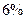Chapter 7, Problem 39P### Principles of Economics 2e

2nd Edition
Steven A. Greenlaw; David Shapiro
ISBN: 9781947172364

#### Solutions

Chapter
Section### Principles of Economics 2e

2nd Edition
Steven A. Greenlaw; David Shapiro
ISBN: 9781947172364
Textbook Problem

# A firm is considering an investment that will earn arate of return. If it were to borrow the money, it would have of payinterest on the loan, but it currently has the cash, so it will But need to borrow. Should the firm make line investment? Show your work.

To determine

Whether the firm should make the investment or not.

Explanation

If the firm involves in the investment, then it would benefit by 6% rate of return and if the firm borrows then the firm is required to pay an interest of 8% on loan. Currently, the firm is having the cash so there is no need to borrow it.

In this case, the firm should not involve in the investment. The borrowing rate is 8% and the firm’s rate of return is 6%. So, the opportunity cost is greater than the returns provided on the investment. The firm is having the abundant amount of cash to commence the investment which provides the return of 6% whereas the cost of borrowing is 8%...

### Still sussing out bartleby?

Check out a sample textbook solution.

See a sample solution

#### The Solution to Your Study Problems

Bartleby provides explanations to thousands of textbook problems written by our experts, many with advanced degrees!

Get Started